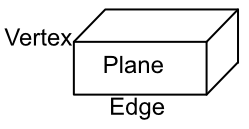Three Dimensions

## Three Dimensions

A three dimensional shape has vertices, edges and planes. A vertex (the plural is vertices) is a point on the object, and a plane is a surface of the object. An edge is the intersection between two planes.## Example 1

How many vertices does a cuboid have?

A vertex is a corner on a three dimensional object. Altogether, a cuboid will have 8 such points.